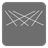Permutation Index
Simple
English

The mission is an idea of przemyslaw.daniel.

Let’s imagine a list of all the permutations of a given set. Every item on the list has it’s index (starting from 1). The task is to calculate the permutation index.

You are given a tuple of integers. It represents one of the permutations of the consecutive integers (starting from 0). You have to return the permutation index of the tuple.

For example

• Input: (1, 2, 0)
• All the consecutive permutations are:
1. (0, 1, 2)
2. (0, 2, 1)
3. (1, 0, 2)
4. (1, 2, 0) !!!
5. (2, 0, 1)
6. (2, 1, 0)
• Output: 4

Example:

```assert permutation_index((2, 0, 1)) == 5
assert permutation_index((2, 1, 3, 0, 4, 5)) == 271
```

Input: One of the permutations of the consecutive integers (a tuple of integers).

Output: The permutation index (an integer).

Precondition:

• sorted(input) == list(range(len(input)))